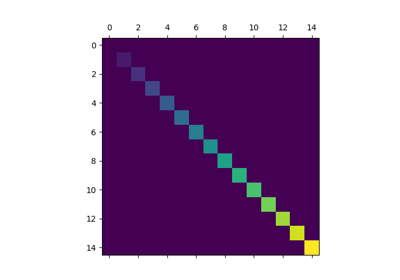# matplotlib.pyplot.matshow¶

matplotlib.pyplot.matshow(A, fignum=None, **kwargs)[source]

Display an array as a matrix in a new figure window.

The origin is set at the upper left hand corner and rows (first dimension of the array) are displayed horizontally. The aspect ratio of the figure window is that of the array, unless this would make an excessively short or narrow figure.

Tick labels for the xaxis are placed on top.

Parameters: A : array-like(M, N) The matrix to be displayed. fignum : None or int or False If None, create a new figure window with automatic numbering. If fignum is an integer, draw into the figure with the given number (create it if it does not exist). If 0 or False, use the current axes if it exists instead of creating a new figure. Note Because of how Axes.matshow tries to set the figure aspect ratio to be the one of the array, strange things may happen if you reuse an existing figure. image : AxesImage **kwargs : imshow arguments

## Examples using matplotlib.pyplot.matshow¶Matshow